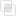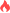Python中random模块的方法整理

Python random2021-09-17 11:08:31862浏览 · 0收藏 · 0评论1、random产生0~1之间的随机小数。

2、randrange在前两个参数范围内产生一个数字。

3、randint在两个参数之间产生一个数字，左右两个参数都可以获得。

choice随机选择列表中的元素并返回。

shuffle打乱列表中元素的顺序。

```import random
r = random.random()  #得到一个0 ~ 1 之间的随机小数
print(r)

r = random.randrange(1,15,2) #打印一个1~15(不包含15)的随机数，步长为2仅能打印(1,3,5,7,9,11,13)
print(r)

r = random.randint(1,10) #产生一个1~10(可包含10)的随机数
print(r)

list1 = ['Tom','Jack','Lily']
r = random.choice(list1)  #choice里放的是列表，随机打印一个列表中的元素
print(r)

pai = ['红桃K','方片A','梅花5','黑桃6']
random.shuffle(pai)  #将列表元素打乱顺序(执行洗牌的动作)
print(pai)

#验证码 大小写字母与数字组合
def func():
code = ''
for i in range(4):
ran1 = str(random.randint(0,9))
ran2 = chr(random.randint(65,90))
ran3 = chr(random.randint(97,122))
r = random.choice([ran1,ran2,ran3])

code +=r
return code
r = func()
print(r)```python切片的使用特性python切片复制列表的本质python定义元组的注意点python if语句的条件测试python使用get()访问字典值python字典遍历所有的键值对怎样进行Python微博爬虫？python字符串的驻留机制是什么python字符串驻留机制的使用范围python中setdefault()的使用python查询键值对是否在字典的三个方法python字典类型的底层实现python集合的底层实现javascript需要编译吗664

How does an anonymous proxy hide your identity1112

What is anonymous proxy510